2021-10-03

Recap

Prev: Descriptive statistics

• stat.desc
• dplyr::across

Next: Exploring assumptions

• Normality
• Skewness and kurtosis
• Homogeneity of variance

Normal distribution

• characterized by the bell-shaped curve
• majority of values lie around the centre of the distribution
• the further the values are from the centre, the lower their frequency
• about 95% of values within 2 standard deviations from the mean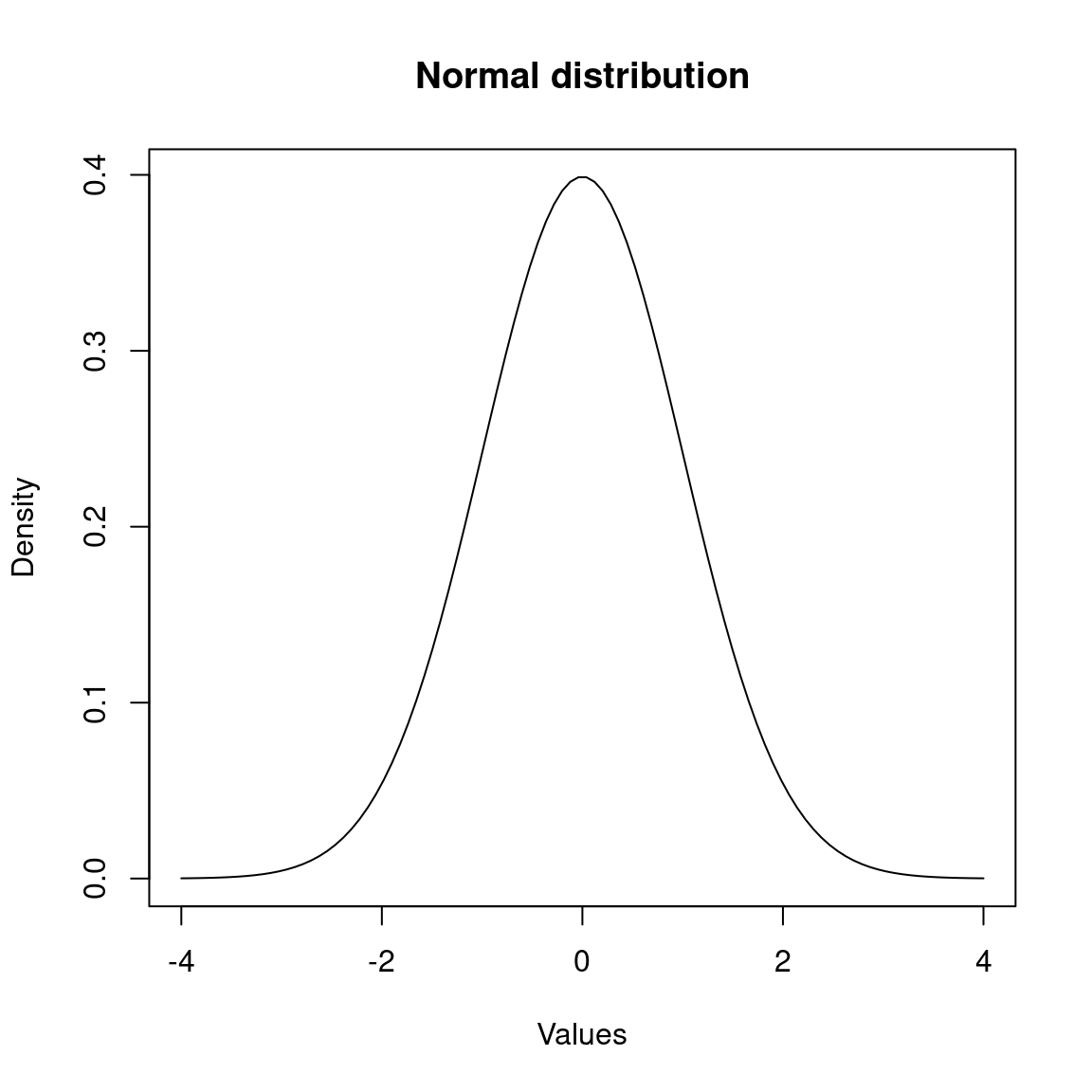Density histogram

palmerpenguins::penguins %>%
ggplot2::ggplot(
aes(x = flipper_length_mm)
) +
ggplot2::geom_histogram(
aes(
y =..density..
)
) +
ggplot2::stat_function(
fun = dnorm,
args = list(
# mean and stddev
# calculations
# omitted here
mean = ...,
sd = ... ),
colour = "black", size = 1)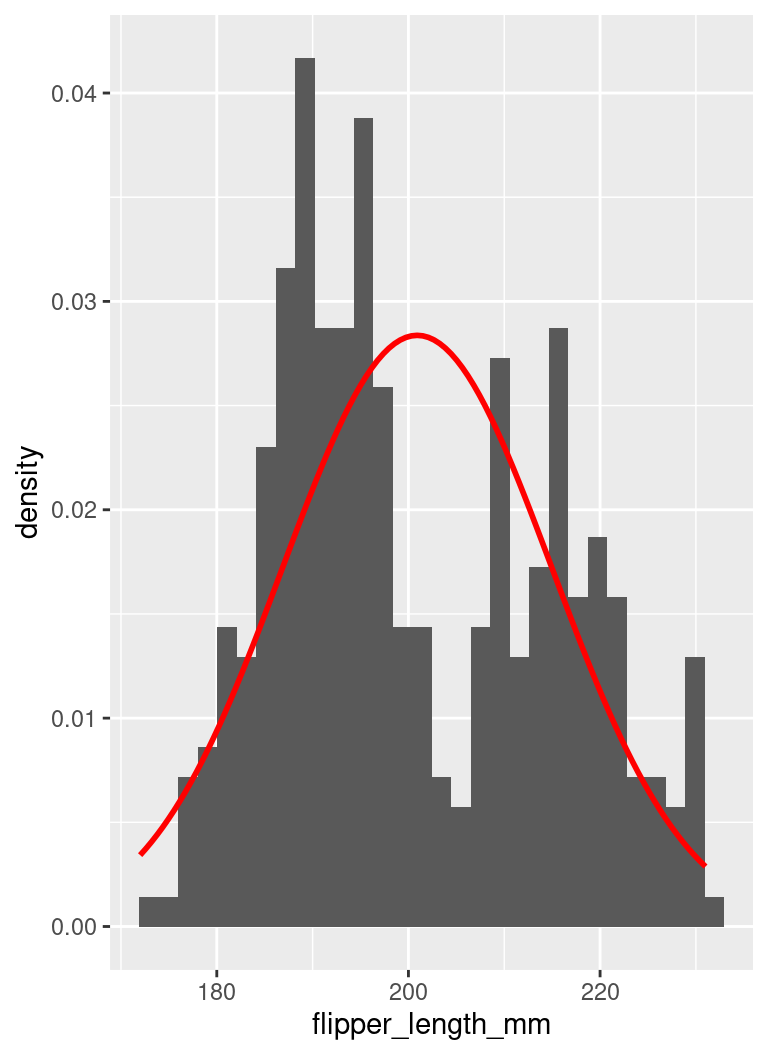Q-Q plot

Values against the cumulative probability of a particular distribution (in this case, normal distribution)

palmerpenguins::penguins %>%
ggplot2::ggplot(
aes(
sample =
flipper_length_mm
)
) +
ggplot2::stat_qq() +
ggplot2::stat_qq_line()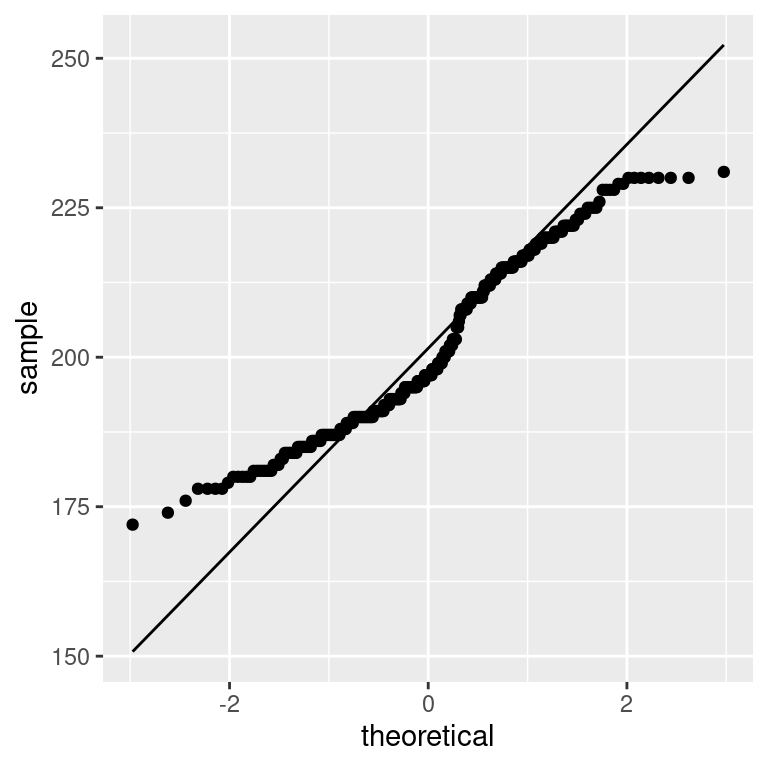Normality

Shapiro–Wilk test compares the distribution of a variable with a normal distribution having same mean and standard deviation

• If significant, the distribution is not normal
• shapiro.test function in stats
• or normtest values in pastecs::stat.desc
palmerpenguins::penguins %>%
dplyr::pull(flipper_length_mm) %>%
stats::shapiro.test()
##
##  Shapiro-Wilk normality test
##
## data:  .
## W = 0.95155, p-value = 3.54e-09

Significance

Most statistical tests are based on the idea of hypothesis testing

• a null hypothesis is set
• the data are fit into a statistical model
• the model is assessed with a test statistic
• the significance is the probability of obtaining that test statistic value by chance

The threshold to accept or reject an hypotheis is arbitrary and based on conventions (e.g., p < .01 or p < .05)

Example: The null hypotheis of the Shapiro–Wilk test is that the sample is normally distributed and p < .01 indicates that the probability of that being true is very low. So, the flipper length of penguins in the Palmer Station dataset is not normally distributed.

Example

The flipper length of Adelie penguins is normally distributed

palmerpenguins::penguins %>%
filter(
) %>%
dplyr::pull(
flipper_length_mm
) %>%
stats::shapiro.test()
##
##  Shapiro-Wilk normality test
##
## data:  .
## W = 0.99339, p-value = 0.72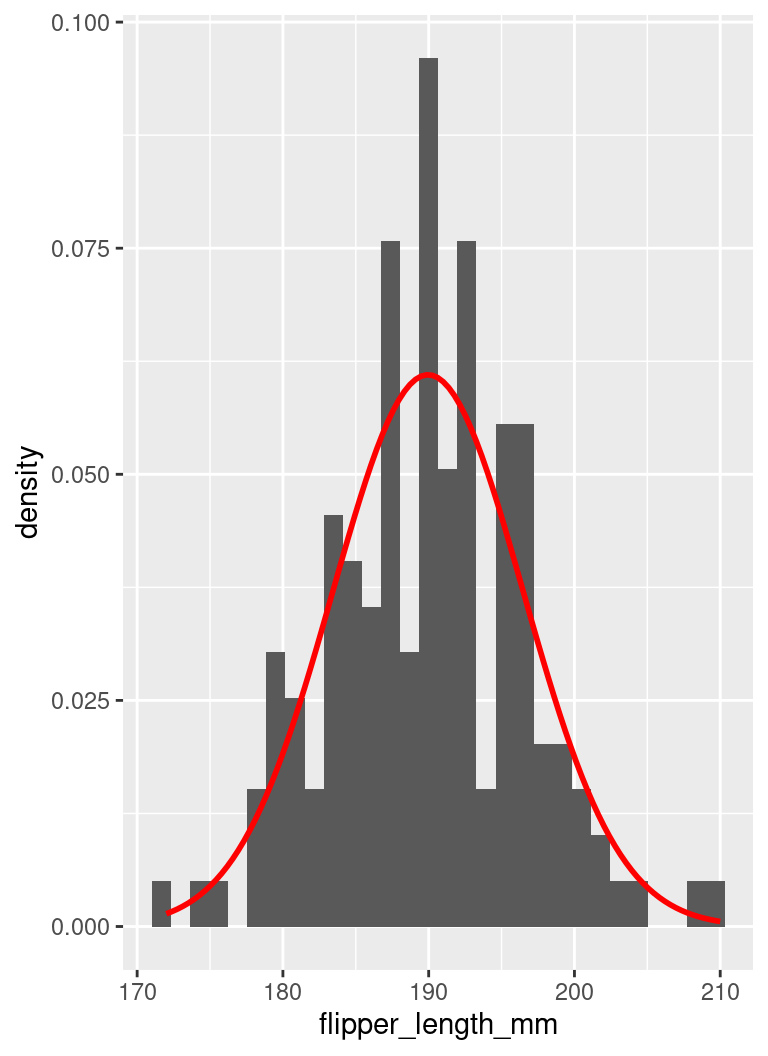Example

The flipper length of Adelie penguins is normally distributed

palmerpenguins::penguins %>%
filter(
) %>%
dplyr::pull(
flipper_length_mm
) %>%
stats::shapiro.test()
##
##  Shapiro-Wilk normality test
##
## data:  .
## W = 0.99339, p-value = 0.72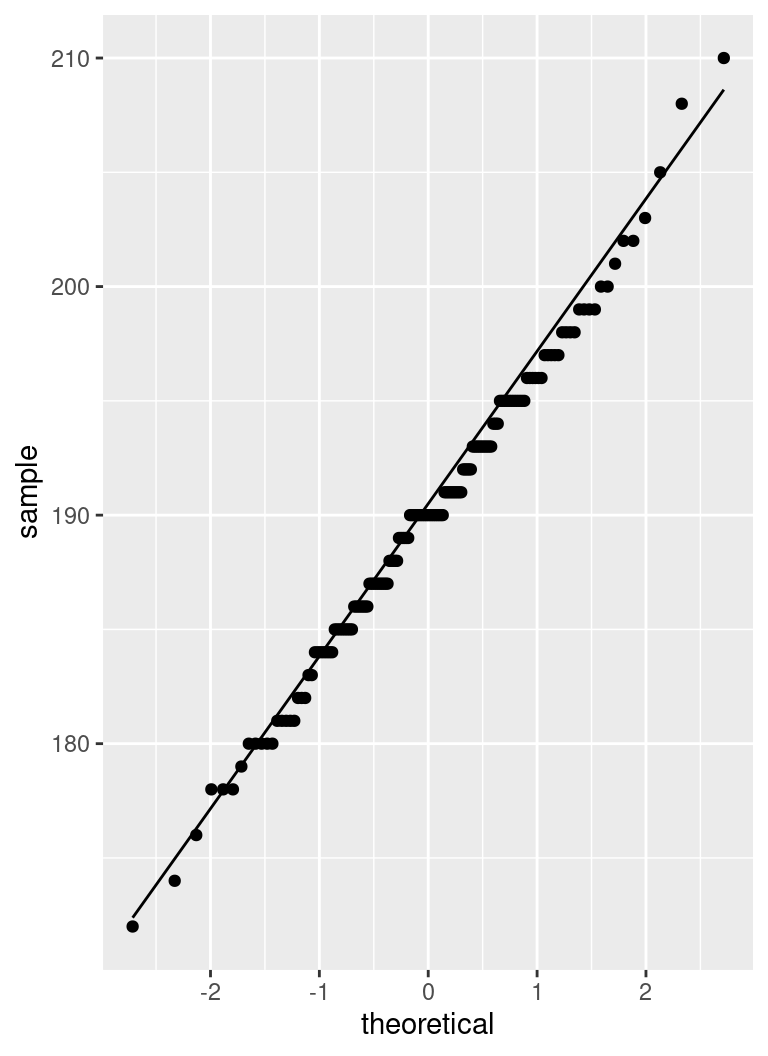Skewness and kurtosis

In a normal distribution, skewness and kurtosis should be zero

• skewness: skewness value indicates
• positive: the distribution is skewed towards the left
• negative: the distribution is skewed towards the right
• kurtosis: kurtosis value indicates
• positive: heavy-tailed distribution
• negative: flat distribution
• skew.2SE and kurt.2SE: skewness and kurtosis divided by 2 standard errors. Therefore
• if > 1 (or < -1) then the stat significant (p < .05)
• if > 1.29 (or < -1.29) then stat significant (p < .01)

Example

Flipper length is not normally distributed

• skewed left (skewness positive, skew.2SE > 1.29)
• flat distribution (kurtosis negative, kurt.2SE < -1.29)
palmerpenguins::penguins %>%
dplyr::select(bill_length_mm, bill_depth_mm, flipper_length_mm) %>%
pastecs::stat.desc(basic = FALSE, desc = FALSE, norm = TRUE)
bill_length_mm bill_depth_mm flipper_length_mm
skewness 0.0526530 -0.1422086 0.3426554
skew.2SE 0.1996290 -0.5391705 1.2991456
kurtosis -0.8931397 -0.9233523 -0.9991866
kurt.2SE -1.6979696 -1.7554076 -1.8995781
normtest.W 0.9748548 0.9725838 0.9515451
normtest.p 0.0000112 0.0000044 0.0000000

Example

• both skew.2SE and kurt.2SE between -1 and 1
palmerpenguins::penguins %>%
dplyr::select(bill_length_mm, bill_depth_mm, flipper_length_mm) %>%
pastecs::stat.desc(basic = FALSE, desc = FALSE, norm = TRUE)
bill_length_mm bill_depth_mm flipper_length_mm
skewness 0.1584764 0.3148847 0.0856093
skew.2SE 0.4014211 0.7976035 0.2168485
kurtosis -0.2285951 -0.1361153 0.2382734
kurt.2SE -0.2913388 -0.1734755 0.3036734
normtest.W 0.9933618 0.9846683 0.9933916
normtest.p 0.7166005 0.0924897 0.7200466

Homogeneity of variance

Levene’s test for equality of variance in different levels

• If significant, the variance is different in different levels
library(car)
palmerpenguins::penguins %>%
car::leveneTest(
body_mass_g ~ species, data = .
)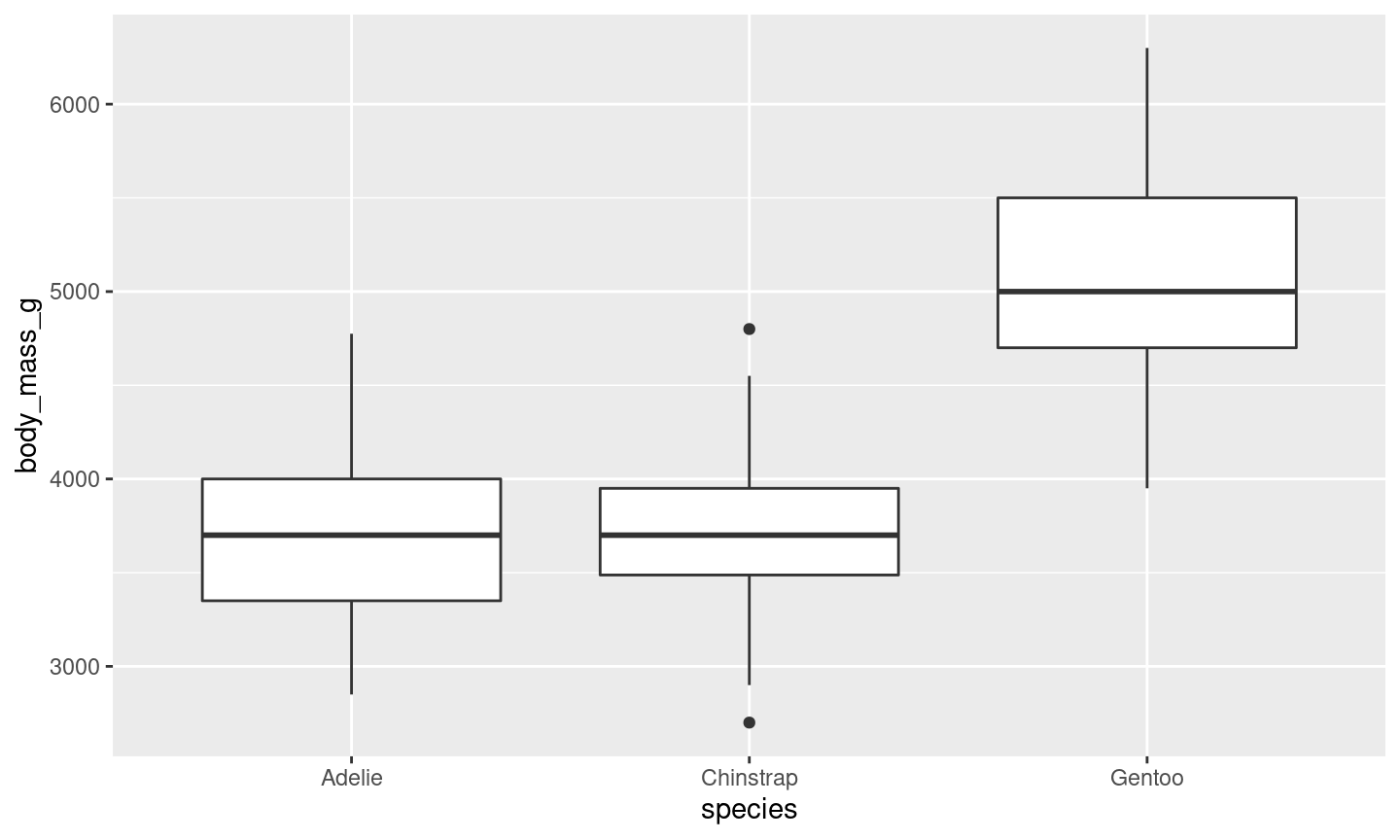## Levene's Test for Homogeneity of Variance (center = median)
##        Df F value   Pr(>F)
## group   2  5.1203 0.006445 **
##       339
## ---
## Signif. codes:  0 '***' 0.001 '**' 0.01 '*' 0.05 '.' 0.1 ' ' 1

Summary

Exploring assumptions

• Normality
• Skewness and kurtosis
• Homogeneity of variance

Next: Practical session

• Data visualisation
• Descriptive statistics
• Exploring assumptions